# Vehicle Electrical and Climate Control Systems

This example shows how to interface the vehicle climate control system with a model of the electrical system to examine the loading effects of the climate control system on the entire electrical system of the car.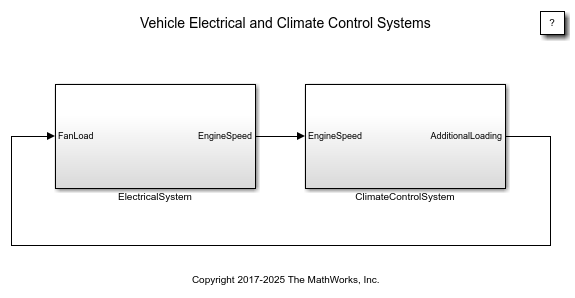Figure 1: Vehicle Electrical and Climate Control System

### The Climate Control System

Double clicking on the ClimateControlSystem subsystem will open the model of the climate control system. Here the user can enter the temperature value they would like the air in the car to reach by double clicking on the USER SETPOINT IN CELSIUS Block and entering the value into the dialog box. The EXTERNAL TEMPERATURE IN CELSIUS can also be set by the user in a similar way. The numerical display on the right hand side of the model shows the reading of a temperature sensor placed behind the driver's head. This is the temperature that the driver should be feeling. When the model is run and the climate control is active, it is this display box whose value changes showing the change of temperature in the car.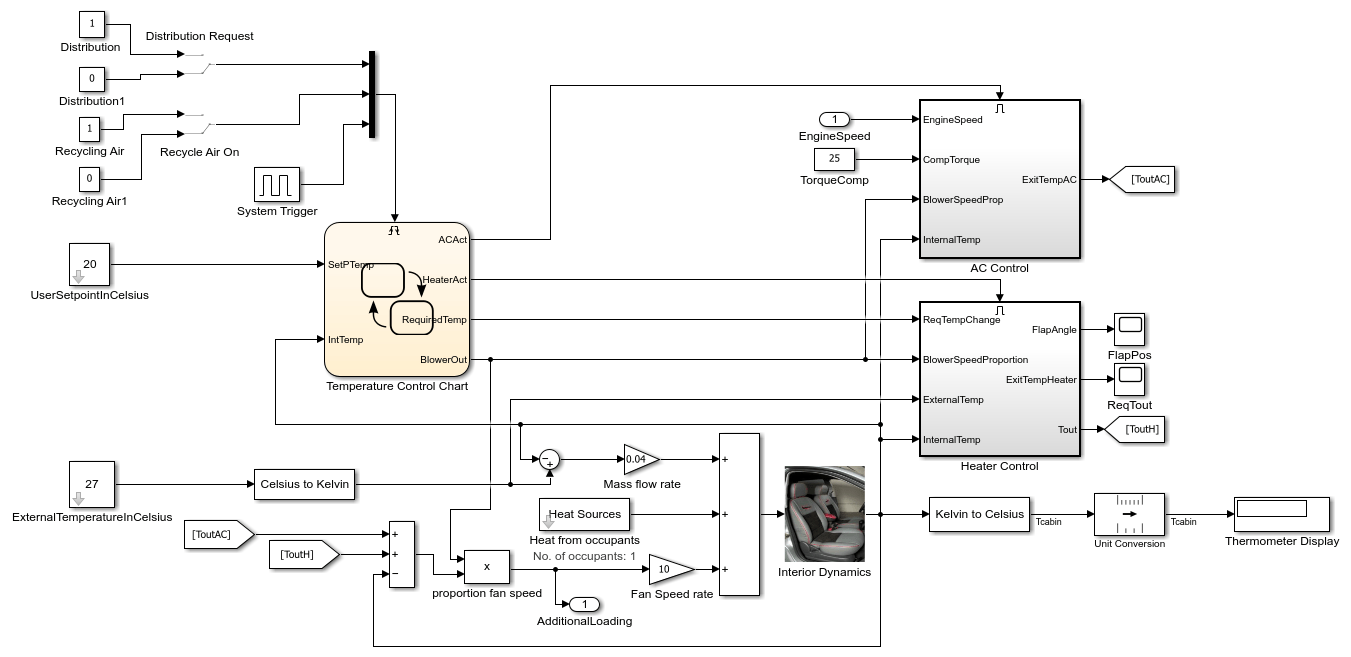Figure 2: The automatic climate control system.

### The Stateflow® Controller

The control of the system is implemented in Stateflow®. Double clicking on the Stateflow chart will show how this supervisory control logic has been formulated.

The Heater_AC state shows that when the user enters a setpoint temperature which greater than the current temperature in the car by at least 0.5 deg C, the heater system will be switched on. The heater will remain active until the current temperature in the car reaches to within 0.5 deg of the setpoint temperature. Similarly, when the user enters a setpoint which is 0.5 deg C (or more) lower than the current car temperature, the Air Conditioner is turned on and stays active until the temperature of the air in the car reaches to within 0.5 deg C of the setpoint temperature. After which, the system will switch off. The dead band of 0.5 deg has been implemented to avoid the problem of continuous switching.

In the Blower State, the larger the difference between the setpoint temperature and the current temperature, the harder the fan blows. This ensures that the temperature will reach the required value in a reasonable amount of time, despite the temperature difference. Once again, when the temperature of the air in the car reaches to within 0.5 deg C of the setpoint temperature, the system will switch off.

The Air Distribution(AirDist) and Recycling Air States(Recyc_Air) are controlled by the two switches that trigger the Stateflow chart. An internal transition has been implemented within these two states to facilitate effective defrosting of the windows when required. When the defrost state is activated, the recycling air is turned off.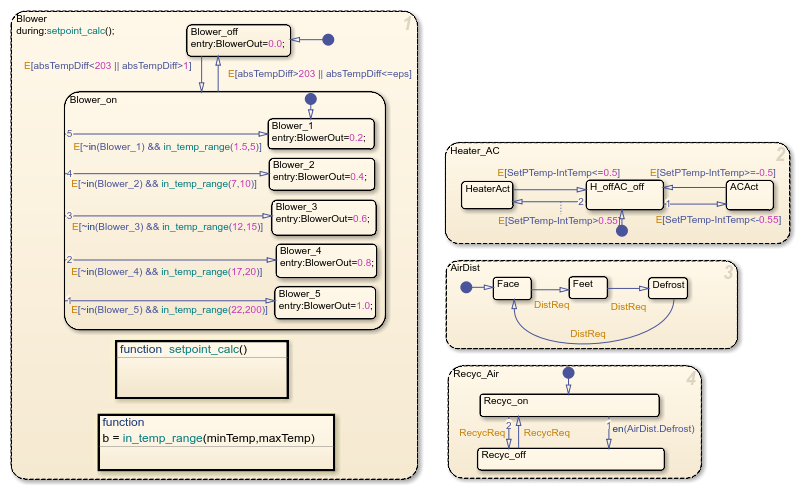Figure 3: The supervisory control logic in Stateflow.

### Heater and Air Conditioner Models

The heater model was built from the equation for a heat exchanger shown below:

`Tout = Ts - (Ts-Tin)e^[(-pi*D*L*hc)/(m_dot*Cp)]`

Where:

• Ts = constant (radiator wall temperature)

• D = 0.004m (channel diameter)

• L = 0.05m (radiator thickness)

• N = 30000 (Number of channels)

• k = 0.026 W/mK = constant (thermal conductivity of air)

• Cp = 1007 J/kgK = constant (specific heat of air)

• Laminar flow (hc = 3.66(k/D) = 23.8 W/m2K )

In addition, the effect of the heater flap is taken into account. Similar to the operation of the blower, the greater the temperature difference between the required setpoint temperature and the current temperature in the car, the more the heater flap is opened and the greater the heating effect.

The Air Conditioner system is one of the two places where the climate control model interfaces with the car's electrical system model. The compressor loads the engine of the car when the A/C system is active. The final temperature to exit from the A/C is calculated as follows:

y*(w*Tcomp) = m_dot*(h4-h1)

Where:

• y = efficiency

• m_dot = mass flow rate

• w = speed of the engine

• Tcomp = compressor torque

• h4, h1 = enthalpy

Here we have bang-bang control of the A/C system where the temperature of the air that exits the A/C is determined by the engine speed and compressor torque.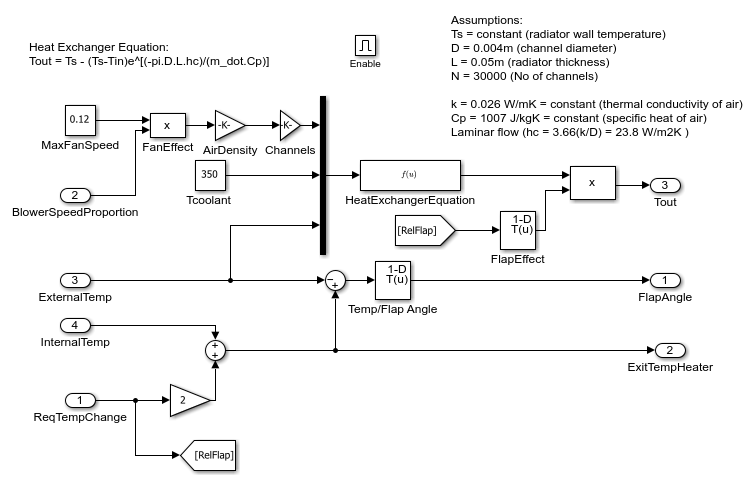Figure 4: Heater control subsystem.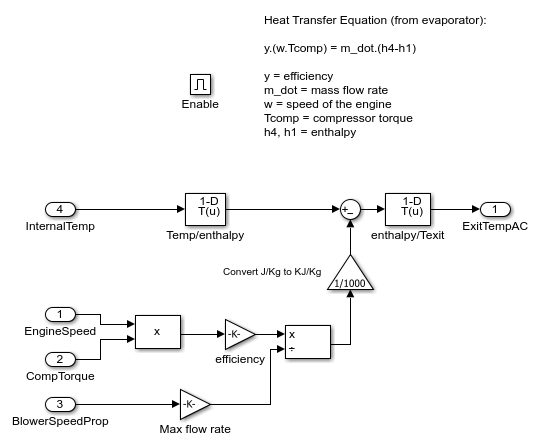Figure 5: A/C control subsystem.

### Heat Transfer in the Cabin

The temperature of the air felt by the driver is affected by all of these factors:

• The temperature of the air exiting the vents

• The temperature of the outside air

• The number of people in the car

These factors are inputs into the thermodynamic model of the interior of the cabin. We take into account the temperature of the air exiting the vents by calculating the difference between the vent air temperature and the current temperature inside the car and multiplying it by the fan speed proportion (mass flow rate). Then 100W of energy is added per person in the car. Lastly, the difference between the temperature of the outside air and the interior air temperature is multiplied by a lesser mass flow rate to account for the air radiating into the car from the outside.

The output of the interior dynamics model is fed to the display block as a measure of the temperature read by a sensor placed behind the driver's head.

### The Electrical System

This electrical system models the car at idle speed. The PID controllers ensure that the car's alternator (modeled by a synchronous machine which has its field current regulated to control the output voltage) is also operating at the required speed. The alternator output is then fed through a 3-phase 6-pulse rectifier bridge to supply the voltage needed to charge the battery which supplies the voltage for the car's DC bus.

The fan used in the climate control system is fed off this DC bus as are the windscreen wipers, radio etc. As the difference between the setpoint temperature and the current temperature in the car drops, so does the fan speed and therefore so does the loading on the DC bus. The inclusion of feedback in the electrical system regulates the DC bus voltage.

The additional model of the car's electrical system allows for the changing of the engine speed. Changing the engine speed shows the effect on the DC bus voltage.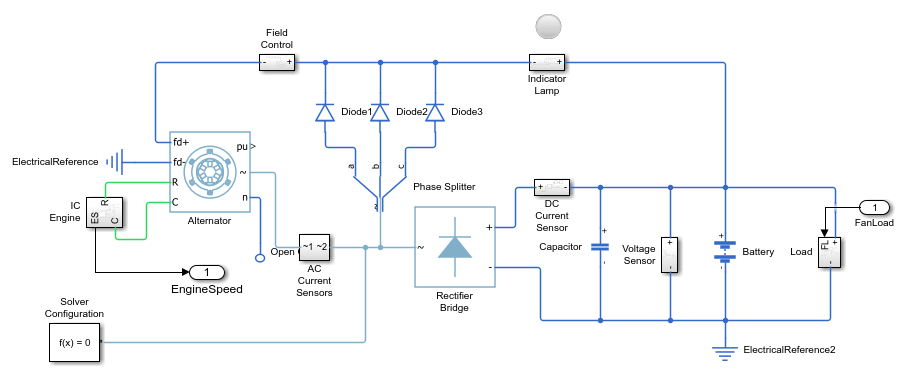Figure 6: The electrical system